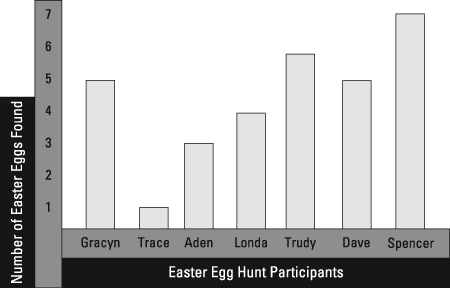##### Praxis Elementary Education For Dummies with Online Practice TestsThe Praxis Elementary Education exam will include questions that ask you to interpret data. This data may come in the form of a bar graph, line graph, circle graph, scatterplot, or box plot.

Bar graphs are a common and effective form of data presentation. They include bars, which are rectangles that correspond to data that is listed vertically. The categories to which those numbers apply are listed horizontally. The categories can be baseball tickets sold for each team in a year, jaywalking incidents in various cities, average hair lengths at universities, and all kinds of other such matters.

• Line graphs are like bar graphs, but line graphs use points connected by lines instead of bars.
• Circle graphs, also known as pie charts, can be used to represent portions.
• Scatterplots use points to represent data involving two measures, such as year and profit. Scatterplot points are a lot like points on the coordinate plane. You can look at the points' patterns and determine how the two measures are related. For example, as one measure increases, the other may tend to increase, decrease, or stay the same. A line of fit can be drawn through approximately the middle of the plotted points to indicate the relationships.
• Box plots are illustrations placed above number lines to show the lowest number, highest number, and median of a set of data. The full range is divided into quartiles, which are quarters of the full ranges of data. Box plots can also indicate outliers, or numbers way outside of the general vicinities of the other numbers.

## Practice question

1. Which of the following statements about the following bar graph is NOT true?A. Dave and Gracyn found the same number of eggs. B. Trace found the lowest number of eggs. C. Londa found more eggs than Trudy. D. Spencer found the most eggs.

1. The correct answer is Choice (C). The bar graph indicates the following numbers of Easter Eggs found for the participants in the hunt.

Trudy found more eggs than Londa, so Londa did not find more eggs than Trudy. Choice (A) is true, so it is an incorrect answer. Dave and Gracyn both found 5 eggs. Choice (B) is an incorrect answer because Trace really did find the lowest number of eggs. He found 1. Everybody else found more than that. Choice (D) also makes a true statement. Spencer found the highest number of eggs.